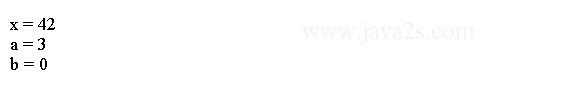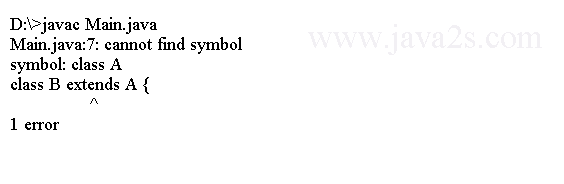# Java Tutorial - Java static final

## Java static keyword

A static class member can be used independently of any object of that class.

A static member that can be used by itself, without reference to a specific instance.

Here shows how to declare `static` method and `static` variable.

```
static int intValue;

static void aStaticMethod(){
}
```

## Restrictions

Methods declared as static have several restrictions:

• They can only call other static methods.
• They must only access static data.
• They cannot refer to this or super in any way.

All instances of the class share the same static variable. You can declare a static block to initialize your static variables. The static block gets only called once when the class is first loaded.

The following example shows a class that has a static method

```
public class Main {
static int a = 3;
static int b;
/*from ww  w. j  ava  2s .  c  om*/
static void meth(int x) {
System.out.println("x = " + x);
System.out.println("a = " + a);
System.out.println("b = " + b);

}

public static void main(String args[]) {
Main.meth(42);
}
}
```

The output:## Example

The following example shows a class that has the static variables.

```
public class Main {
static int a = 3;
static int b;
}
```

We can reference the static variables defined above as follows:

```Main.a
```

The following example shows a class that has a static initialization block.

```
public class Main {

static int a = 3;

static int b;

static {
System.out.println("Static block initialized.");
b = a * 4;
}
}
```

## Java final keyword

A final variable cannot be modified. You must initialize a final variable when it is declared. A final variable is essentially a constant.

## Final variables

```
public class Main {
final int FILE_NEW = 1;
final int FILE_OPEN = 2;
}
```

## Prevent overriding

Methods declared as final cannot be overridden.

```
class Base {/*from w  w w  . ja  v a  2  s. c o m*/
final void meth() {
System.out.println("This is a final method.");
}
}

class B extends A {
void meth() { // ERROR! Can't override.

System.out.println("Illegal!");

}
}
```

If you try to compile the code above, the following error will be generated by the compiler.